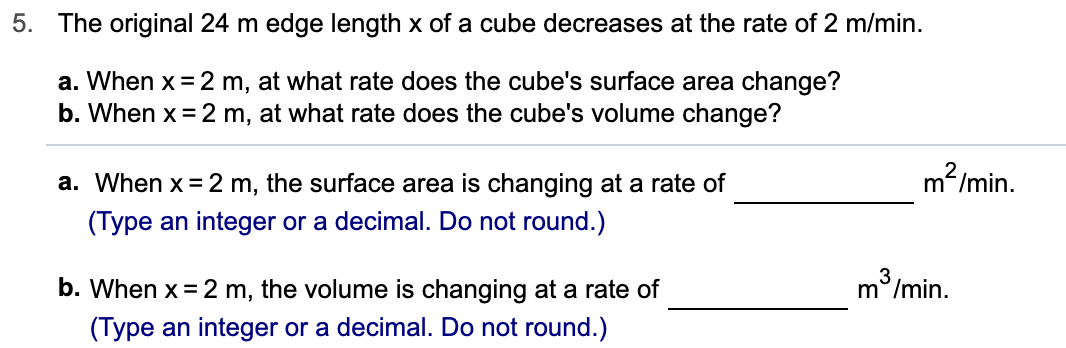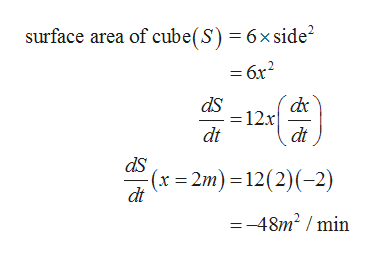# 5. The original 24 m edge length x of a cube decreases at the rate of 2 m/min.a. When x 2 m, at what rate does the cube's surface area change?b. When x 2 m, at what rate does the cube's volume change?m2/min.a. When x 2 m, the surface area is changing at a rate of(Type an integer or a decimal. Do not round.)m /min.b. When x 2 m, the volume is changing at a rate of(Type an integer or a decimal. Do not round.)

Question

Can you help me step by step?help_outlineImage Transcriptionclose5. The original 24 m edge length x of a cube decreases at the rate of 2 m/min. a. When x 2 m, at what rate does the cube's surface area change? b. When x 2 m, at what rate does the cube's volume change? m2/min. a. When x 2 m, the surface area is changing at a rate of (Type an integer or a decimal. Do not round.) m /min. b. When x 2 m, the volume is changing at a rate of (Type an integer or a decimal. Do not round.) fullscreen
check_circle

Step 1

According to the given information:

Length of the cube = ‘x’ m

Rate at which the length decreases (dx/dt) = -2m/min

Step 2

For part (a) it is needed to calculate the rate of ...help_outlineImage Transcriptionclosesurface area of cube(S) 6x side2 =6x2 dS = 12x dt dt ds (x 2m) 12(2) (-2) dt =-48m2 /min fullscreen

### Want to see the full answer?

See Solution

#### Want to see this answer and more?

Solutions are written by subject experts who are available 24/7. Questions are typically answered within 1 hour.*

See Solution
*Response times may vary by subject and question.
Tagged in

### Derivative